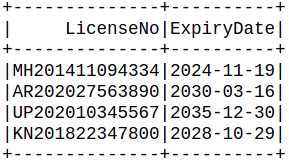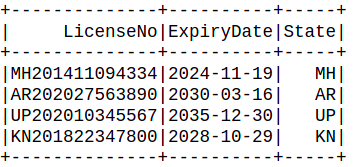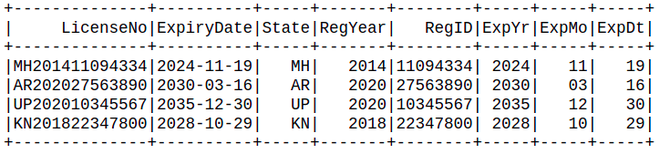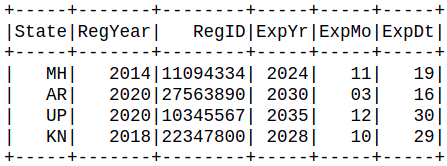# How to check for a substring in a PySpark dataframe ?

• Last Updated : 18 Jul, 2021

In this article, we are going to see how to check for a substring in PySpark dataframe.

Substring is a continuous sequence of characters within a larger string size. For example, “learning pyspark” is a substring of “I am learning pyspark from GeeksForGeeks”. Let us look at different ways in which we can find a substring from one or more columns of a PySpark dataframe.

Attention geek! Strengthen your foundations with the Python Programming Foundation Course and learn the basics.

To begin with, your interview preparations Enhance your Data Structures concepts with the Python DS Course. And to begin with your Machine Learning Journey, join the Machine Learning - Basic Level Course

Creating Dataframe for demonstration:

## Python

 `# importing module``import` `pyspark`` ` `# importing sparksession from pyspark.sql module``from` `pyspark.sql ``import` `SparkSession`` ` `# creating sparksession and giving an app name``spark ``=` `SparkSession.builder.appName(``'sparkdf'``).getOrCreate()`` ` ` ` `# Column names for the dataframe``columns ``=` `[``"LicenseNo"``, ``"ExpiryDate"``]`` ` `# Row data for the dataframe``data ``=` `[``    ``(``"MH201411094334"``, ``"2024-11-19"``),``    ``(``"AR202027563890"``, ``"2030-03-16"``),``    ``(``"UP202010345567"``, ``"2035-12-30"``),``    ``(``"KN201822347800"``, ``"2028-10-29"``),``]`` ` `# Create the dataframe using the above values``reg_df ``=` `spark.createDataFrame(data``=``data,``                               ``schema``=``columns)`` ` `# View the dataframe``reg_df.show()`

Output:In the above dataframe, LicenseNo is composed of 3 information, 2-letter State Code + Year of registration + 8 digit registration number.

## Method 1: Using DataFrame.withColumn()

The DataFrame.withColumn(colName, col) can be used for extracting substring from the column data by using pyspark’s substring() function along with it.

Syntax: DataFrame.withColumn(colName, col)

Parameters:

• colName: str, name of the new column
• col: str, a column expression for the new column

Returns a new DataFrame by adding a column or replacing the existing column that has the same name.

We will make use of the pyspark’s substring() function to create a new column “State” by extracting the respective substring from the LicenseNo column.

Syntax: pyspark.sql.functions.substring(str, pos, len)

Example 1: For single columns as substring.

## Python

 `from` `pyspark.sql.functions ``import` `substring`` ` `reg_df.withColumn(``  ``'State'``, substring(``'LicenseNo'``, ``1``, ``2``)``).show()`

Output:Here, we have created a new column “State” where the substring is taken from “LicenseNo” column. (1, 2) indicates that we need to start from the first character and extract 2 characters from the “LicenseNo” column.

Example 2: For multiple columns as substring

Extracting State Code as ‘State’, Registration Year as ‘RegYear’, Registration ID as ‘RegID’, Expiry Year as ‘ExpYr’, Expiry Date as ‘ExpDt’, Expiry Month as ‘ExpMo’.

## Python

 `from` `pyspark.sql.functions ``import` `substring`` ` `reg_df \``.withColumn(``'State'`  `, substring(``'LicenseNo'` `, ``1``, ``2``)) \``.withColumn(``'RegYear'``, substring(``'LicenseNo'` `, ``3``, ``4``)) \``.withColumn(``'RegID'`  `, substring(``'LicenseNo'` `, ``7``, ``8``)) \``.withColumn(``'ExpYr'`  `, substring(``'ExpiryDate'``, ``1``, ``4``)) \``.withColumn(``'ExpMo'`  `, substring(``'ExpiryDate'``, ``6``, ``2``)) \``.withColumn(``'ExpDt'`  `, substring(``'ExpiryDate'``, ``9``, ``2``)) \``.show()`

Output:The above code demonstrates how withColumn() method can be used multiple times to get multiple substring columns. Each withColumn() method adds a new column in the dataframe. It is worth noting that it also retains the original columns as well.

## Method 2: Using substr inplace of substring

Alternatively, we can also use substr from column type instead of using substring.

Syntax:pyspark.sql.Column.substr(startPos, length)

Returns a Column which is a substring of the column that starts at ‘startPos’ in byte and is of length ‘length’ when ‘str’ is Binary type.

Example: Using substr

## Python

 `from` `pyspark.sql.functions ``import` `col`` ` `reg_df \``.withColumn(``'State'`  `, col(``'LicenseNo'` `).substr(``1``, ``2``)) \``.withColumn(``'RegYear'``, col(``'LicenseNo'` `).substr(``3``, ``4``)) \``.withColumn(``'RegID'`  `, col(``'LicenseNo'` `).substr(``7``, ``8``)) \``.withColumn(``'ExpYr'`  `, col(``'ExpiryDate'``).substr(``1``, ``4``)) \``.withColumn(``'ExpMo'`  `, col(``'ExpiryDate'``).substr(``6``, ``2``)) \``.withColumn(``'ExpDt'`  `, col(``'ExpiryDate'``).substr(``9``, ``2``)) \``.show()`

Output:The substr() method works in conjunction with the col function from the spark.sql module. However, more or less it is just a syntactical change and the positioning logic remains the same.

## Method 3: Using DataFrame.select()

Here we will use the select() function to substring the dataframe.

Syntax: pyspark.sql.DataFrame.select(*cols)

Example: Using DataFrame.select()

## Python

 `from` `pyspark.sql.functions ``import` `substring`` ` `reg_df.select(``  ``substring(``'LicenseNo'` `, ``1``, ``2``).alias(``'State'``)  ,``  ``substring(``'LicenseNo'` `, ``3``, ``4``).alias(``'RegYear'``),``  ``substring(``'LicenseNo'` `, ``7``, ``8``).alias(``'RegID'``)  ,``  ``substring(``'ExpiryDate'``, ``1``, ``4``).alias(``'ExpYr'``)  ,``  ``substring(``'ExpiryDate'``, ``6``, ``2``).alias(``'ExpMo'``)  ,``  ``substring(``'ExpiryDate'``, ``9``, ``2``).alias(``'ExpDt'``)  ,``).show()`

Output:## Method 4: Using ‘spark.sql()’

The spark.sql() method helps to run relational SQL queries inside spark itself. It allows the execution of relational queries, including those expressed in SQL using Spark.

Syntax: spark.sql(expression)

Example: Using ‘spark.sql()’

## Python

 `reg_df.createOrReplaceTempView(``"reg_view"``)`` ` `reg_df2 ``=` `spark.sql(``'''``SELECT ``  ``SUBSTR(LicenseNo, 1, 3)  AS State,``  ``SUBSTR(LicenseNo, 3, 4)  AS RegYear,``  ``SUBSTR(LicenseNo, 7, 8)  AS RegID,``  ``SUBSTR(ExpiryDate, 1, 4) AS ExpYr,``  ``SUBSTR(ExpiryDate, 6, 2) AS ExpMo,``  ``SUBSTR(ExpiryDate, 9, 2) AS ExpDt``FROM reg_view;``'''``)`` ` `reg_df2.show()`

Output:Here, we can see the expression used inside the spark.sql() is a relational SQL query. We can use the same in an SQL query editor as well to fetch the respective output.

## Method 5: Using spark.DataFrame.selectExpr()

Using selectExpr() method is a way of providing SQL queries, but it is different from the relational ones’. We can provide one or more SQL expressions inside the method. It takes one or more SQL expressions in a String and returns a new DataFrame

Syntax: selectExpr(exprs)

Example: Using spark.DataFrame.selectExpr().

## Python

 `from` `pyspark.sql.functions ``import` `substring`` ` `reg_df.selectExpr(``  ``'LicenseNo'``,``  ``'ExpiryDate'``,``  ``'substring(LicenseNo , 1, 2) AS State'`   `,``  ``'substring(LicenseNo , 3, 4) AS RegYear'` `,``  ``'substring(LicenseNo , 7, 8) AS RegID'`   `,``  ``'substring(ExpiryDate, 1, 4) AS ExpYr'`   `,``  ``'substring(ExpiryDate, 6, 2) AS ExpMo'`   `,``  ``'substring(ExpiryDate, 9, 2) AS ExpDt'`   `,``).show()`

Output:In the above code snippet, we can observe that we have provided multiple SQL expressions inside the selectExpr() method. Each of these expressions resemble a part of the relational SQL query that we write. We also preserved the original columns by mentioning them explicitly.

My Personal Notes arrow_drop_up